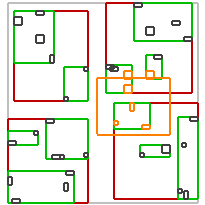#Boost C++ Libraries

...one of the most highly regarded and expertly designed C++ library projects in the world.

### Queries

Queries returns `Value`s which meets some predicates. Currently supported are three types of predicates:

• spatial predicates - spatial conditions that must be met by stored Value and some Geometry,
• distance predicates - distance conditions that must be met by stored Value and some Geometry,
• user-defined unary predicate - function, function object or lambda expression checking user-defined condition.

For example queries may be used to retrieve Values:

• intersecting some area but not within other area,
• are nearest to some point,
• overlapping a box and has user-defined property.
##### Performing a query

There are three ways to perform a query presented below. All of them returns `Value`s intersecting some region defined as a `Box`.

Method call

```std::vector<Value> returned_values;
Box box_region(...);
rt.query(bgi::intersects(box_region), std::back_inserter(returned_values));
```

Function call

```std::vector<Value> returned_values;
Box box_region(...);
index::query(rt, bgi::intersects(box_region), std::back_inserter(returned_values));
```

Use of pipe operator generating a range

```Box box_region(...);
BOOST_FOREACH(Value & v, rt | index::adaptors::queried(bgi::intersects(box_region)))
; // do something with v
```
##### Spatial predicates

Queries using spatial predicates returns `Value`s which are related somehow to some Geometry - box, polygon, etc. Names of spatial predicates correspond to names of Boost.Geometry algorithms. Examples of some basic queries may be found in tables below. The query region and result `Value`s are orange.

intersects(Box)

covered_by(Box)

disjoint(Box)

overlaps(Box)

within(Box)intersects(Ring)

intersects(Polygon)

intersects(MultiPolygon)To use a spatial predicate one may use one of the functions defined in `boost::geometry::index` namespace.

```rt.query(index::intersects(box), std::back_inserter(result));
rt.query(index::covered_by(box), std::back_inserter(result));
rt.query(index::disjont(box), std::back_inserter(result));
rt.query(index::overlaps(box), std::back_inserter(result));
rt.query(index::within(box), std::back_inserter(result));
```

All spatial predicates may be negated, e.g.:

```rt.query(!index::intersects(box), std::back_inserter(result));
// the same as
rt.query(index::disjoint(box), std::back_inserter(result));
```
##### Distance predicates
###### Nearest neighbours queries

Nearest neighbours queries returns `Value`s which are closest to some point in space. Additionally it is possible to define how the distance to the `Value` should be calculated. The example of knn query is presented below. 5 `Value`s nearest to some point are orange.###### k nearest neighbours

There are three ways of performing knn queries. Following queries returns `k` `Value`s closest to some point in space. For `Box`es `Indexable`s the distance to the nearest point is calculated by default.

Method call

```std::vector<Value> returned_values;
Point pt(...);
rt.query(index::nearest(pt, k), std::back_inserter(returned_values));
```

Function call

```std::vector<Value> returned_values;
Point pt(...);
index::query(rt, index::nearest(pt, k), std::back_inserter(returned_values));
```

Use of `operator |`

```Point pt(...);
BOOST_FOREACH(Value & v, rt | index::adaptors::queried(index::nearest(pt, k)))
; // do something with v
```
##### User-defined unary predicate

The user may pass a `UnaryPredicate` - function, function object or lambda expression taking const reference to Value and returning bool. This object may be passed to the query in order to check if `Value` should be returned by the query. To do it one may use `index::satisfies()` function like on the example below:

```bool is_red(Value const& v)
{
return v.is_red();
}

struct is_red_o
{
template <typename Value>
bool operator()(Value const& v)
{
return v.is_red();
}
}

// ...

rt.query(index::intersects(box) && index::satisfies(is_red),
std::back_inserter(result));

rt.query(index::intersects(box) && index::satisfies(is_red_o()),
std::back_inserter(result));

#ifndef BOOST_NO_CXX11_LAMBDAS
rt.query(index::intersects(box) && index::satisfies([](Value const& v) { return v.is_red(); }),
std::back_inserter(result));
#endif
```

`satisfies()` may be negated, e.g.:

```bool is_red(Value const& v) { return v.is_red(); }
bool is_not_red(Value const& v) { return !v.is_red(); }

// ...

rt.query(index::intersects(box) && index::satisfies(is_red),
std::back_inserter(result));
// the same as
rt.query(index::intersects(box) && !index::satisfies(is_not_red),
std::back_inserter(result));
```
##### Passing a set of predicates

It's possible to use some number of predicates in one query by connecting them with `operator&&` e.g. ```Pred1 && Pred2 && Pred3 && ...```.

These predicates are connected by logical AND. Passing all predicates together not only makes possible to construct advanced queries but is also faster than separate calls because the tree is traversed only once. Traversing is continued and `Value`s are returned only if all predicates are met. Predicates are checked left-to-right so placing most restrictive predicates first should accelerate the search.

```rt.query(index::intersects(box1) && !index::within(box2),
std::back_inserter(result));

rt.query(index::intersects(box1) && !index::within(box2) && index::overlaps(box3),
std::back_inserter(result));
```

Of course it's possible to connect different types of predicates together.

```index::query(rt, index::nearest(pt, k) && index::within(b), std::back_inserter(returned_values));

BOOST_FOREACH(Value & v, rt | index::adaptors::queried(index::nearest(pt, k) && index::covered_by(b)))
; // do something with v
```
##### Inserting query results into the other R-tree

There are several ways of inserting Values returned by a query to the other R-tree container. The most basic way is creating a temporary container for Values and insert them later.

```namespace bgi = boost::geometry::index;
typedef std::pair<Box, int> Value;
typedef bgi::rtree< Value, bgi::linear<32, 8> > RTree;

RTree rt1;
/* some inserting into the tree */

std::vector<Value> result;
rt1.query(bgi::intersects(Box(/*...*/)), std::back_inserter(result));
RTree rt2(result.begin(), result.end());
```

However there are better ways. One of these methods is mentioned in the "Creation and modification" section. The insert iterator may be passed directly into the query.

```RTree rt3;
rt1.query(bgi::intersects(Box(/*...*/))), bgi::inserter(rt3));
```

If you like Boost.Range you'll appreciate the third option. You may pass the result Range directly into the constructor.

```RTree rt4(rt1 | bgi::adaptors::queried(bgi::intersects(Box(/*...*/)))));
```